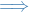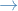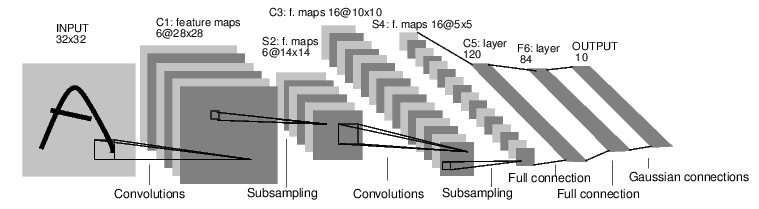# PyTorch

## Overview

• Tensor computation (like numpy) with strong GPU acceleration
• Deep Neural Networks built on tape-based autograd system
• Tape-based autograd = keeps track of computation you perform with variables
• Allows for dynamic neural networks, i.e. change the way the net works arbitrarily with zero lag or overhead

If you ever want a nice introduction, just head over here.

That tutorial shows how to implement a simple feed-forward neural network using:

• Numpy
• PyTorch (without autograd)
• TensorFlow (for comparison to static computation graphs)
• PyTorch (with autograd)

## Syntax

In you get most the stuff you do in numpy.

import numpy as np
import matplotlib as plt
import torch


### Tensor

torch.Tensor(3, 5)  # unitialized

torch.rand(3, 5)  # randomized between 0 and 1


### In-place operations

Any operations that mutats a tensor in-place is post-fixed with an _. For example: y.add_(x) will add x to y, changing y.

x = torch.Tensor([[1, 2, 3], [4, 5, 6]])
y = torch.Tensor([[1, 2, 3], [4, 5, 6]])

y


### Numpy interface

a = np.ones(5)
b = torch.from_numpy(a)

all([x == y for x, y in zip(a, b)])  # <= points to the same data!


### CUDA

if torch.cuda.is_available():
print(":)")
else:
print(":(")


• Found in the autograd package
• Define-by-run frameworkbackprop is defined by how you run your code
• Contrast to frameworks like TensorFlow and Theano, which constructs and compiles a computation graph before run (these use symbolic differentiation)

### Variable

• autograd.Variable
• Finished with computationcall .backward() and have all the gradients be computed automatically
• Interconnected with autograd.Function

### Overview

• Variable and Function build up an acyclic graph, which encodes the complete history of computation
• Each Variable has a .creator attribute which references a Function that has created the Variable (user-created has None)
• If Variable is a scalar > no arguments to =.backward() necessary
• If Variable has multiple elements > need to specify =grad_output argument that is a tensor of matching shape.

Process for creating a computation and performing backprop is as follows:

1. Create Variable instances
2. Do computations
3. Call .backward() on result of computation
4. View the derivative of the computation you just called .backward() on wrt. to some Variable by accessing the .grad attribute on this Variable.
from torch.autograd import Variable

x

y = x + 2
y

y.creator

z = y * y * 3
out = z.mean()
z, out

out.backward()


## Neural Networks

• Constructed using torch.nn package
• Depends on autograd to define models and differentiate them
• nn.Module contains layers, and a method forward(input) that returns the output

### Example: MNIST Convolutional Network

Let's classify some digits!#### Define the network

import torch
import torch.nn as nn
import torch.nn.functional as F

class Net(nn.Module):

def __init__(self):
super(Net, self).__init__()
# 1 input image channel, 6 output channels, 5x5 square convolution
# kernel
self.conv1 = nn.Conv2d(1, 6, 5)  # 1 input channel = greyscale
self.conv2 = nn.Conv2d(6, 16, 5)
# an affine operation: y = Wx + b
self.fc1 = nn.Linear(16 * 5 * 5, 120)
self.fc2 = nn.Linear(120, 84)
self.fc3 = nn.Linear(84, 10)

def forward(self, x):
# Max pooling over a (2, 2) window
x = F.max_pool2d(F.relu(self.conv1(x)), (2, 2))
# If the size is a square you can only specify a single number
x = F.max_pool2d(F.relu(self.conv2(x)), 2)
# reshaping, in this case flatten it
x = x.view(-1, self.num_flat_features(x))

# Affine operations
x = F.relu(self.fc1(x))
x = F.relu(self.fc2(x))
x = self.fc3(x)
return x

def num_flat_features(self, x):
size = x.size()[1:]  # all dimensions except the batch dimension
num_features = 1
for s in size:
num_features *= s
return num_features

net = Net()
net


That is… awesome.

params = list(net.parameters())
params.size()

input = Variable(torch.randn(1, 1, 32, 32))  # 32 because ?
out = net(input)
out

net.zero_grad()
out.backward(torch.randn(1, 10))  # initialize weights randomly


=torch.nn only supports mini-matches, not single sample.

For example, nn.Conv2d will take in a 4D Tensor of n_samples x n_channels x height x width.

If you have a single sample, just use input.unsqueeze(0) to add a fake batch dimension.

##### Loss function
output = net(input)
target = Variable(torch.range(1, 10))  # dummy target
criterion = nn.MSELoss()  # mean-squared-error

loss = criterion(output, target)
loss


Now, if you follow loss in the backward direction, using it’s .creator attribute, you will see a graph of computations that looks like this:

input -> conv2d -> relu -n> maxpool2d -> conv2d -> relu -> maxpool2d -> view -> linear -> relu -> linear -> relu -> linear -> MSELoss -> loss

So, when we call loss.backward(), the whole graph is differentiated w.r.t. the loss, and all Variables in the graph will have their .grad Variable accumulated with the gradient.

loss.creator.previous_functions

##### Backprop
net.zero_grad()  # zeros gradient buffers of all parameters

loss.backward()


Again, awesome!

##### Updating the weights

For simplicity, we'll simply do the standard Stochastic Gradient Descent (SDG).

learning_rate = 0.01
for f in net.parameters():

params  # first filter or feature-map of conv1


However, we'll probably like to use different update rules. We can find these in the optim package.

import torch.optim as optim

optimizer = optim.SGD(net.parameters(), lr=0.01)

output = net(input)
loss = criterion(output, target)
loss.backward()
optimizer.step()  # does the update

params


### Example: Linear regression

import numpy as np
import matplotlib.pyplot as plt

from sklearn.datasets import make_regression
from sklearn.model_selection import train_test_split
from sklearn.metrics import log_loss

import torch
import torch.nn as nn

RANDOM_SEED = 42
np.random.seed(RANDOM_SEED)

# Hyperparameters
input_size = 1
output_size = 1
epochs_n = 60
alpha = 0.001

# Dataset
X, y = make_regression(n_features=1, random_state=RANDOM_SEED)
X, y = X.astype(np.float32), y.astype(np.float32)
X_train, X_test, y_train, y_test = train_test_split(
X, y, random_state=RANDOM_SEED)

# Linear regression model
class LinearRegression(nn.Module):
def __init__(self, input_size, output_size):
super(LinearRegression, self).__init__()
self.linear = nn.Linear(input_size, output_size)

def forward(self, x):
out = self.linear(x)
return out

reg_model = LinearRegression(input_size, output_size)

# Training the model
for epoch in range(epochs_n):
# Convert numpy array to Variable
inputs = Variable(torch.from_numpy(X_train))
outputs = Variable(torch.from_numpy(y_train))

# Forward
predictions = reg_model.forward(inputs)

# Backward
loss = (predictions - outputs).pow(2).sum()
loss.backward()

# Update

predictions_test = reg_model.forward(Variable(torch.from_numpy(X_test))).data.numpy()
print(np.square((y_test - predictions_test)).sum())
print(predictions_test.shape)

plt.plot(X_test, predictions_test, label="pred")
plt.scatter(X_test, y_test, label="true", color="g")
plt.legend()
plt.show()


### Example: Feed Forward Neural Network on linear regression

import numpy as np
import matplotlib.pyplot as plt

from sklearn.datasets import make_regression
from sklearn.model_selection import train_test_split
from sklearn.metrics import log_loss

import torch
import torch.nn as nn
import torch.nn.functional as F

RANDOM_SEED = 42
np.random.seed(RANDOM_SEED)

# Hyperparameters
input_size = 1
output_size = 1
epochs_n = 100
batch_size = 20
alpha = 0.001

# Dataset
X, y = make_regression(n_features=input_size, random_state=RANDOM_SEED)
X, y = X.astype(np.float32), y.astype(np.float32)
X_train, X_test, y_train, y_test = train_test_split(
X, y, random_state=RANDOM_SEED)

class FeedForwardNeuralNetwork(nn.Module):
def __init__(self, input_size, layer_sizes):
self.layers = [nn.Linear(input_size, layer_sizes)]
for i in range(len(layer_sizes) - 1):
l = nn.Linear(*layer_sizes[i:i+2])
self.layers.append(l)

def forward(self, x):
for l in self.layers[:-1]:
x = F.sigmoid(l(x))

x = self.layers[-1](x)
return x

for l in self.layers:

model = FeedForwardNeuralNetwork(input_size, [20, 30, 1])

# Training the model
for epoch in range(epochs_n):
# Convert numpy array to Variable
indices = np.random.randint(X_train.shape, size=batch_size)
inputs = Variable(torch.from_numpy(X_train[indices]))
outputs = Variable(torch.from_numpy(y_train[indices]))

# Forward
predictions = model.forward(inputs)

# Backward
loss = (predictions - outputs).pow(2).sum()
loss.backward()

# Update
for l in model.layers:

predictions_test = model.forward(Variable(torch.from_numpy(X_test))).data.numpy()
print(np.square((y_test - predictions_test)).sum())
print(predictions_test.shape)

plt.scatter(X_test, predictions_test, label="pred")
plt.scatter(X_test, y_test, label="true", color="g")
plt.legend()
plt.show()


### Example: Recurrent Neural Network on linear regression

import numpy as np
import matplotlib.pyplot as plt

from sklearn.datasets import make_regression
from sklearn.model_selection import train_test_split
from sklearn.metrics import log_loss

import torch
import torch.nn as nn
import torch.nn.functional as F

from progress.bar import Bar

RANDOM_SEED = 42
np.random.seed(RANDOM_SEED)

# Hyperparameters
input_size = 1
output_size = 1
window_size = 5
hidden_size = 24
# In this particular problem, increasing epochs_n might give you nan
# predictions I believe this is due to the extrapolating nature of the problem,
# where we are looking ahead when trying to make predictions on the test data.
epochs_n = 100
alpha = 0.001

# Dataset
X, y = make_regression(n_features=1, random_state=RANDOM_SEED)
X, y = X.astype(np.float32), y.astype(np.float32)
X_train, X_test, y_train, y_test = train_test_split(
X, y, random_state=RANDOM_SEED
)

class RNN(nn.Module):
def __init__(self, input_size, output_size, hidden_size):
super(RNN, self).__init__()

self.W_hx = nn.Linear(input_size, hidden_size)
self.W_hh = nn.Linear(hidden_size, hidden_size)
self.W_hy = nn.Linear(hidden_size, output_size)

# hidden state

def forward(self, X):
"""X is a sequence of observations"""
for x in X:
self.h = self.W_hh(self.h) + self.W_hx(x.resize(x.size(), 1))
self.h = F.tanh(self.h)

return self.W_hy(self.h)

model = RNN(input_size, output_size, hidden_size)

X_train = torch.from_numpy(X_train)
y_train = torch.from_numpy(y_train)
X_test = torch.from_numpy(X_test)
y_test = torch.from_numpy(y_test)

def plot_rnn_predictions(model, X, y=None, window_size=3, **plot_kwargs):
xs = []
preds = []

if y is not None:
ys = []

for i in range(1, X_test.size() + 1):
end_idx = i
start_idx = max(end_idx - window_size, 0)
input_seq = Variable(X_test[start_idx: end_idx])
pred = model.forward(input_seq)
xs.append(X_test[end_idx - 1])
preds.append(pred.data.squeeze())

if y is not None:
ys.append(y[end_idx - 1])

plt.scatter(xs, preds, **plot_kwargs)

if y is not None:
plt.scatter(xs, ys, label="true", color="g")

plot_rnn_predictions(model, X_test, window_size=window_size,
label="pre-train", color="r")

losses = []
bar = Bar("Epoch", max=epochs_n)
for epoch in range(epochs_n):
# progress
bar.next()

# on second thought: we would probably get better performance,
# or rather faster convergence, if we trained on entire dataset
# and predict some output after each step. This would allows us
# to fit the hidden layer h on EACH STEP, rather than after
# each window_size sequence-step.
# ACTUALLY this might pose an issue when using PyTorch, as the
# autograd would backpropagate aaaaall the way back.
# Thus, we ought to do exactly what we do now, but of course
# run through all of the time-steps.
end_idx = np.random.randint(1, X_train.size() + 1)
start_idx = max(end_idx - window_size, 0)
inputs = Variable(X_train[start_idx: end_idx])
output = y_train[end_idx - 1]

# Forward
pred = model.forward(inputs)

# Backward
loss = (pred - output).pow(2)
losses.append(loss.data.squeeze())
loss.backward(retain_variables=True)

# Update

plot_rnn_predictions(model, X_test, y=y_test, window_size=window_size,
label="predictions", color="b")
plt.suptitle("Recurrent Neural Network")
plt.title("Linear regression with window size of %d" % window_size)
plt.legend()
plt.show()

# setting the title for some reason messed up the previous plot
# and I'm not bothered to fix this right now :)
# Hence => don't add title!
plt.scatter(range(len(losses)), losses)
plt.title("Losses over %d epochs" % epochs_n)
plt.xlabel("epoch")
plt.ylabel("loss")
plt.show()

# progress.bar does not add a newline to the end, so we fix
print()


## Appendix A: Definitions

Affine operation
operation on the affine space, which is a generalization of the Euclidean space that are independent of the concepts of distance and measure of angles, keeping only the properties related to parallelism and ratio of lengths for parallel line segments. Note: Euclidean space is an affine space.